# Python读取Excel的文本框

## 基本需求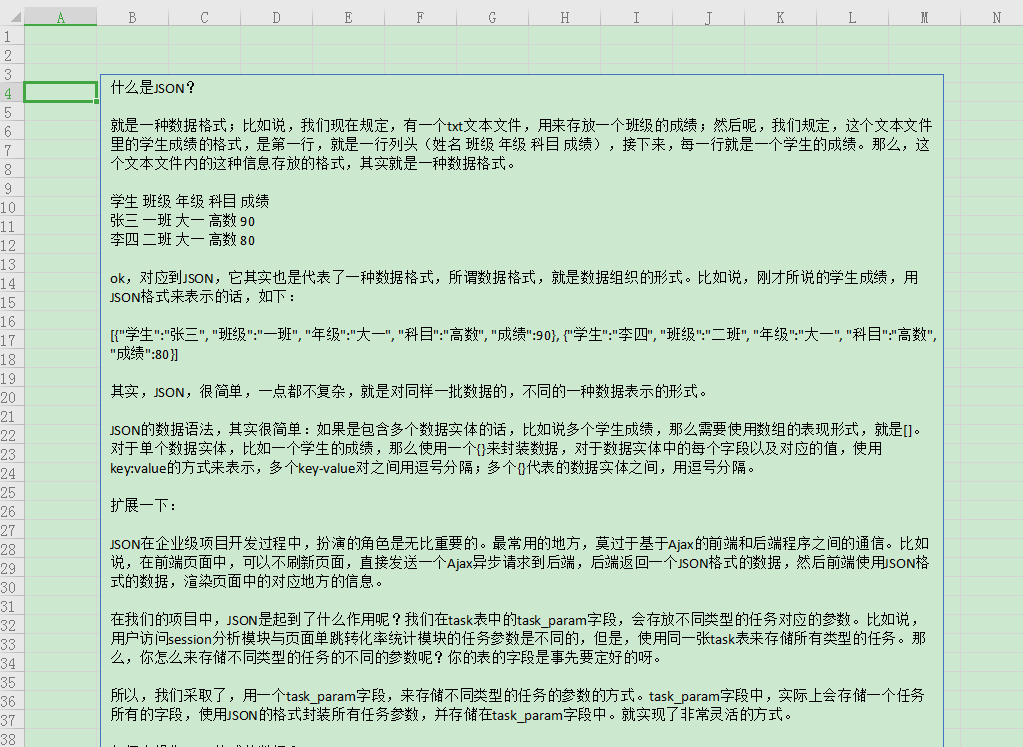## 处理代码

xlsx文件的本质是xml格式的压缩包，解压文件做xml解析提取出相应的数据即可。

``````import re
import os
import shutil
from zipfile import ZipFile

tempdir = tempfile.gettempdir()
basename = os.path.basename(xlsx_file)
xml_names = []
with ZipFile(xlsx_file) as zip_file:
for name in zip_file.namelist():
if name.startswith("xl/drawings/drawing"):
zip_file.extract(name, tempdir)
destname = f"{tempdir}/{name}"
xml_names.append(destname)
result = []
for xml_name in xml_names:
with open(xml_name, encoding="utf-8") as f:
lines = re.findall("<a:p>(.*?)</a:p>", text)
for line in lines:
runs = re.findall("<a:t>(.*?)</a:t>", line)
result.append("".join(runs).replace('&lt;', '<').replace(
'&gt;', '>').replace('&amp;', '&'))
return "\n".join(result)
``````

``````result = read_xlsx_textbox_text("test.xlsx")
print(result)
``````

``````什么是JSON？

ok，对应到JSON，它其实也是代表了一种数据格式，所谓数据格式，就是数据组织的形式。比如说，刚才所说的学生成绩，用JSON格式来表示的话，如下：

[{"学生":"张三", "班级":"一班", "年级":"大一", "科目":"高数", "成绩":90}, {"学生":"李四", "班级":"二班", "年级":"大一", "科目":"高数", "成绩":80}]

JSON的数据语法，其实很简单：如果是包含多个数据实体的话，比如说多个学生成绩，那么需要使用数组的表现形式，就是[]。对于单个数据实体，比如一个学生的成绩，那么使用一个{}来封装数据，对于数据实体中的每个字段以及对应的值，使用key:value的方式来表示，多个key-value对之间用逗号分隔；多个{}代表的数据实体之间，用逗号分隔。
...
``````

## 读取xls文件的文本框内容

``````import win32com.client as win32

excel_app = win32.gencache.EnsureDispatch('Excel.Application')
try:
wb = excel_app.Workbooks.Open(xls_file)
xlsx_file = xls_file+"x"
wb.SaveAs(xlsx_file, FileFormat=51)
finally:
excel_app.Quit()
``````

``````print(read_xls_textbox_text(r"E:\tmp\test2.xls"))
``````

``````我们的数据从哪里来？

...
``````

## xls格式批量转xlsx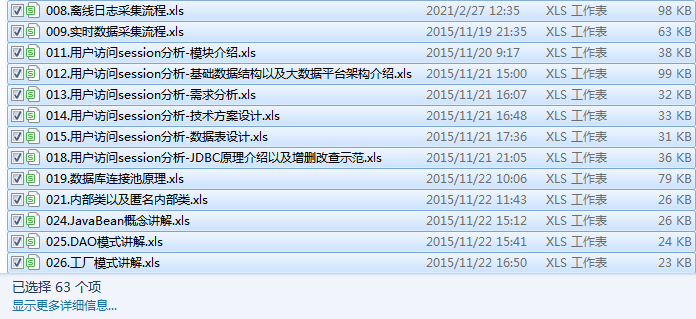``````import win32com.client as win32  # 导入模块
from pathlib import Path
import os

def format_conversion(xls_path, output_path):
if not os.path.exists(output_path):
os.makedirs(output_path)
excel_app = win32.gencache.EnsureDispatch('Excel.Application')
try:
for filename in Path(xls_path).glob("[!~]*.xls"):
dest_name = f"{output_path}/{filename.name}x"
wb = excel_app.Workbooks.Open(filename)
wb.SaveAs(dest_name, FileFormat=51)
print(dest_name, "保存完成")
finally:
excel_app.Quit()
``````

``````excel_path = r"F:\excel文档"
output_path = r"E:\tmp\excel"
format_conversion(excel_path, output_path)
``````

``````E:\tmp\excel/008.离线日志采集流程.xlsx 保存完成
E:\tmp\excel/009.实时数据采集流程.xlsx 保存完成
E:\tmp\excel/011.用户访问session分析-模块介绍.xlsx 保存完成
E:\tmp\excel/012.用户访问session分析-基础数据结构以及大数据平台架构介绍.xlsx 保存完成
E:\tmp\excel/013.用户访问session分析-需求分析.xlsx 保存完成
E:\tmp\excel/014.用户访问session分析-技术方案设计.xlsx 保存完成
E:\tmp\excel/015.用户访问session分析-数据表设计.xlsx 保存完成
E:\tmp\excel/018.用户访问session分析-JDBC原理介绍以及增删改查示范.xlsx 保存完成
E:\tmp\excel/019.数据库连接池原理.xlsx 保存完成
...
``````

## 批量提取xlsx文件的文本框文本

``````from pathlib import Path

xlsx_path = r"E:\tmp\excel"
for filename in Path(xlsx_path).glob("[!~]*.xlsx"):
filename = str(filename)
destname = filename.replace(".xlsx", ".txt")
print(filename, destname)
with open(destname, "w") as f:
f.write(txt)
``````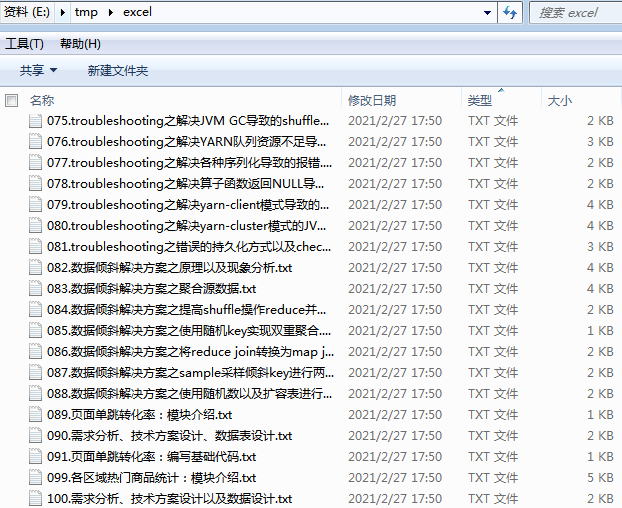## 需求升级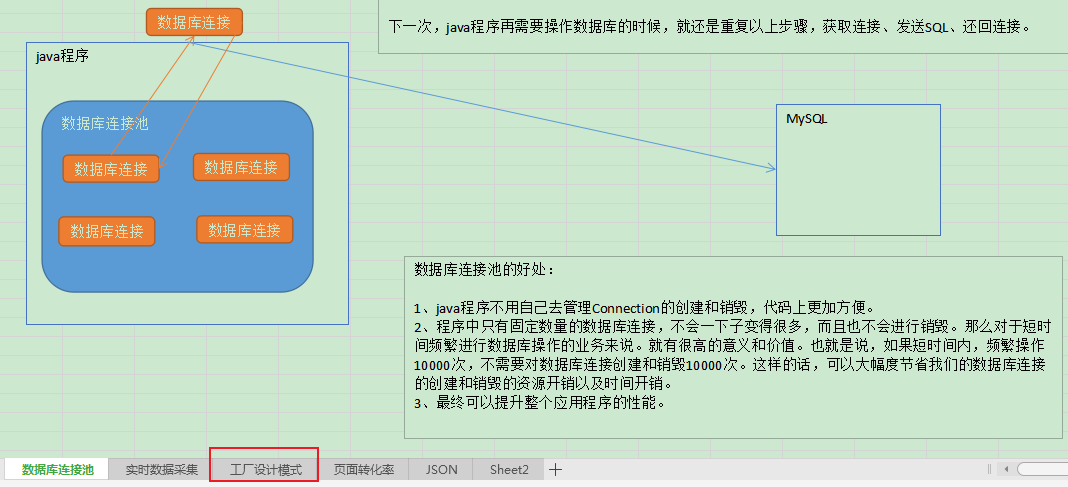``````from zipfile import ZipFile
from pathlib import Path
import shutil
import os
import tempfile
import re

xlsx_file = "test3.xlsx"

tempdir = tempfile.gettempdir()
basename = os.path.basename(xlsx_file)
xml_names = []
sheets_names = None
ids = []
with ZipFile(xlsx_file) as zip_file:
for name in zip_file.namelist():
if name.startswith("xl/drawings/drawing"):
zip_file.extract(name, tempdir)
destname = f"{tempdir}/{name}"
xml_names.append(destname)
elif name == "xl/workbook.xml":
zip_file.extract(name, tempdir)
sheets_names = f"{tempdir}/{name}"
elif name.startswith("xl/worksheets/_rels/sheet"):
tmp = name.lstrip("xl/worksheets/_rels/sheet")
ids.append(int(tmp[:tmp.find(".")])-1)
print(xml_names, sheets_names, ids)
``````

``````['C:\\Users\\Think\\AppData\\Local\\Temp/xl/drawings/drawing1.xml', 'C:\\Users\\Think\\AppData\\Local\\Temp/xl/drawings/drawing2.xml', 'C:\\Users\\Think\\AppData\\Local\\Temp/xl/drawings/drawing3.xml', 'C:\\Users\\Think\\AppData\\Local\\Temp/xl/drawings/drawing4.xml', 'C:\\Users\\Think\\AppData\\Local\\Temp/xl/drawings/drawing5.xml'] C:\Users\Think\AppData\Local\Temp/xl/workbook.xml [0, 1, 2, 4, 5]
``````

``````with open(sheets_names, encoding="utf-8") as f:
sheet_names = re.findall(
'<sheet .*?name="([^"]+)" .*?/>', text)
tmp = []
for inx in ids:
tmp.append(sheet_names[inx])
sheet_names = tmp
sheet_names
``````

``````['JSON', '数据库连接池', '实时数据采集', '工厂设计模式', '页面转化率']
``````

``````result = {}
for sheet_name, xml_name in zip(sheet_names, xml_names):
with open(xml_name, encoding="utf-8") as f:
lines = re.findall("<a:p>(.*?)</a:p>", xml)
tmp = []
for line in lines:
runs = re.findall("<a:t>(.*?)</a:t>", line)
tmp.append("".join(runs).replace('&lt;', '<').replace(
'&gt;', '>').replace('&amp;', '&'))
result[sheet_name] = "\n".join(tmp)
result
``````

``````{'JSON': '什么是JSON？....',
'数据库连接池': 'java程序\n数据库连接\n数据库连接\n数据库连接\nMySQL...',
'实时数据采集': '...实时数据，通常都是从分布式消息队列集群中读取的，比如Kafka....',
'工厂设计模式': '如果没有工厂模式，可能会出现的问题：....',
'页面转化率': '用户行为分析大数据平台\n\n页面单跳转化率，....'}
``````

## 分别读取每个sheet对应文本框文本

``````import re
import os
from zipfile import ZipFile
import tempfile

tempdir = tempfile.gettempdir()
basename = os.path.basename(xlsx_file)
xml_names = []
sheets_names = None
ids = []
with ZipFile(xlsx_file) as zip_file:
for name in zip_file.namelist():
if name.startswith("xl/drawings/drawing"):
zip_file.extract(name, tempdir)
destname = f"{tempdir}/{name}"
xml_names.append(destname)
elif name == "xl/workbook.xml":
zip_file.extract(name, tempdir)
sheets_names = f"{tempdir}/{name}"
elif name.startswith("xl/worksheets/_rels/sheet"):
tmp = name.lstrip("xl/worksheets/_rels/sheet")
ids.append(int(tmp[:tmp.find(".")])-1)
with open(sheets_names, encoding="utf-8") as f:
sheet_names = re.findall(
'<sheet .*?name="([^"]+)" .*?/>', text)
tmp = []
for inx in ids:
tmp.append(sheet_names[inx])
sheet_names = tmp
result = {}
for sheet_name, xml_name in zip(sheet_names, xml_names):
with open(xml_name, encoding="utf-8") as f:
lines = re.findall("<a:p>(.*?)</a:p>", xml)
tmp = []
for line in lines:
runs = re.findall("<a:t>(.*?)</a:t>", line)
tmp.append("".join(runs).replace('&lt;', '<').replace(
'&gt;', '>').replace('&amp;', '&'))
result[sheet_name] = "\n".join(tmp)
if combine:
return "\n".join(result.values())
return result
``````

``````result = read_xlsx_textbox_text("test3.xlsx")
print(result)
``````

## 批量提取文本框文本分sheet单独保存

``````from pathlib import Path
import os

xlsx_path = r"E:\tmp\excel"
for filename in Path(xlsx_path).glob("[!~]*.xlsx"):
dest = filename.with_suffix("")
if not os.path.exists(dest):
os.mkdir(dest)
filename = str(filename)
print(filename, dest)
for txtname, txt in result.items():
with open(f"{dest}/{txtname}", "w") as f:
f.write(txt)
print(f"\t{dest}/{txtname}")
``````

## 使用Python调用VBA解决需求

``````import win32com.client as win32

if app is None:
excel_app = win32.gencache.EnsureDispatch('Excel.Application')
else:
excel_app = app
wb = excel_app.Workbooks.Open(excel_file)
result = {}
for sht in wb.Sheets:
if sht.Shapes.Count == 0:
continue
lines = []
for shp in sht.Shapes:
try:
text = shp.TextFrame2.TextRange.Text
lines.append(text)
except Exception as e:
pass
result[sht.Name] = "\n".join(lines)
if app is None:
excel_app.Quit()
if combine:
return "\n".join(result.values())
return result
``````

``````result = read_excel_textbox_text(r'F:\jupyter\test\提取word图片\test3.xlsx')
print(result)
``````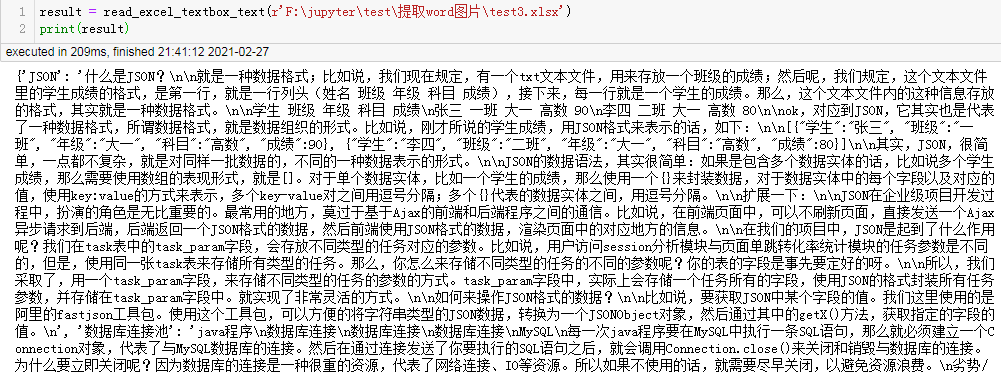``````from pathlib import Path
import os

xlsx_path = r"E:\tmp\excel"
app = win32.gencache.EnsureDispatch('Excel.Application')
try:
for filename in Path(xlsx_path).glob("[!~]*.xls"):
dest = filename.with_suffix("")
if not os.path.exists(dest):
os.mkdir(dest)
filename = str(filename)
print(filename, dest)
for txtname, txt in result.items():
with open(f"{dest}/{txtname}", "w") as f:
f.write(txt)
print(f"\t{dest}/{txtname}")
finally:
app.Quit()
``````

## 使用xlwings解决需求

``````import xlwings as xw

wb = app.books.open(r'test3.xlsx')
for sht in wb.sheets:
print("-------------", sht.name)
for shp in sht.shapes:
if hasattr(shp, 'text') and shp.text:
print(shp.text)
wb.close()
app.quit()
``````

pip install xlwings -U

## 总结

• VBA是excel应用直接支持的API，代码编写起来相对很简单，但执行效率低下。苹果电脑无法使用VBA，可以使用xlwings已经封装好的方法实现。
• 直接解析xml文件，需要对excel的存储格式较为了解，编码起来很费劲，但是执行效率极高。